# The PageRank calculations

## Why doesn't the spreadsheet give the same result as those other "PageRank explanation sites" do?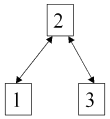Very simple set-up
It does - eventually.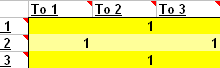How to specify a very simple set-up
Take the very simple set-up to the left. Page2 links to two sub-pages - and they both link back. This is specified on the spreadsheet shown to the right.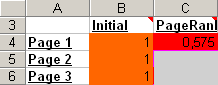First calculation

First we calculate Page1's PageRank. Page1 is pointed to from Page2, which points to two pages. So the formula becomes:

PR(Page1)   =   (1-d) + d (PR(Page2)/2)   =   (1 - 0.85) + 0.85 (1/2)   =   0.575

So we turn our attention to Page2 - and our trouble starts. Page2 is pointed to from Page1 and Page3. But we have just calculated a new PageRank for Page1. So which one do we use in this calculation - the initial value or the new value? And when we calculate Page3's PageRank, do we use Page2's new PageRank?.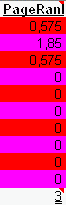First iteration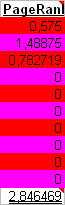First iteration, skewed method
The column to the left shows the result with calculations based on the previous iteration - the right column shows the "skewed" way of doing it, where the most recent results are used.

There are several things to be noted here:

• In the long run the values will converge and become the same.
• The "skewed" method will converge faster.
• With the "skewed" method Page1 and 3 have different ranks - even though they ought to be identical (they will be - after enough iterations).
• With the "skewed" method the site's total PageRank is not exactly 3 (it will be - after enough iterations).

So which method is used by Google? Nobody outside the Googleplex knows - and it doesn't matter since the values end up the same.